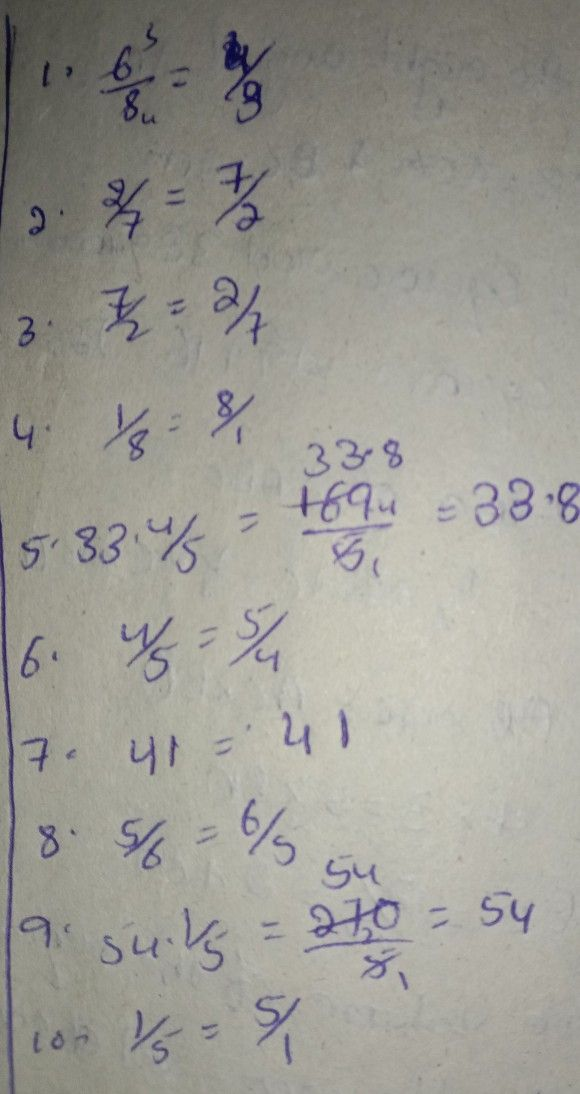Symbol
Problem$E$ Learning Task $2$ Give the reciprocal of the given fractions below. Write your answer in your notebook. 1. $6$ $6$ $\dfrac {2} {7}$ 8 $2$ 7. $\dfrac {1} {8}$ $\dfrac {7} {2}$ $3$ 8. $33\dfrac {4} {5}$ $4-$ $41$ 9. $\dfrac {4} {5}$ $\dfrac {5} {6}$ $5$ $10$ $54\dfrac {1} {5}$ $\dfrac {1} {5}$ $A$
1st-6th grade
Other
Search count: 113
SolutionQanda teacher - ruchiraStudent
thankyou